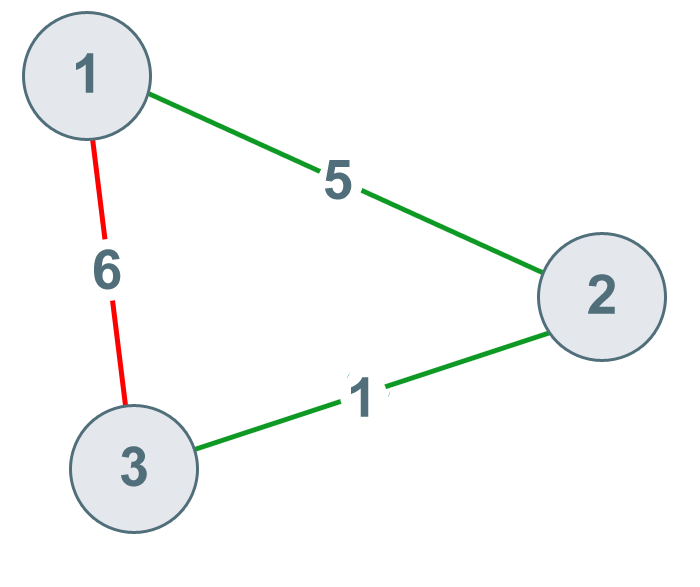There are `N` cities numbered from `1` to `N`.

You are given connections, where each `connections[i] = [city1, city2, cost]` represents the cost to connect `city1` and `city2together`. (A connection is `bidirectional`: connecting `city1` and `city2` is the same as connecting `city2` and `city1`.)

Return the minimum cost so that for every pair of cities, there exists a path of connections (possibly of length `1`) that connects those two cities together. The cost is the sum of the connection costs used. If the task is impossible, return `-1`.

NOTE: The answer may be 0, i.e. removing the entire string.

### Examples

#### Example 1:

##### Input: `N = 3`, `connections = [[1,2,5],[1,3,6],[2,3,1]]`#### Example 2:

##### Input: `N = 4`, `connections = [[1,2,3],[3,4,4]]`##### Explanation:

There is no way to connect all cities even if all edges are used.

#### Constrains:

`1 <= N <= 10000`

`1 <= connections.length <= 10000`

`1 <= connections[i], connections[i] <= N`

`0 <= connections[i] <= 10^5`

`connections[i] != connections[i]`

### Solution:

Try to connect cities with minimum cost, then find a small cost edge first, if two cities connected by the edge do not have the same ancestor, then union them.

When the number of unions equal to `1`, all cities is connected.

Time Complexity: `O(mlogm + mlogN)`. sort takes `O(mlogm)`. find takes `O(logN)`. With path compression and unino by weight, amatorize O(1).

Space: `O(N)`.